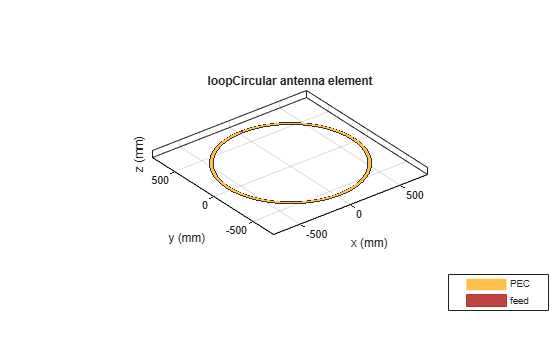# loopCircular

Create circular loop antenna

## Description

The `loopCircular` object is a planar circular loop antenna on the X-Y plane.

The thickness of the loop is related to the diameter of an equivalent cylinder loop by the equation

`$t=2d=4r$`

, where:

• d is the diameter of equivalent cylindrical loop

• r is the radius of equivalent cylindrical loop

For a given cylinder radius, use the `cylinder2strip` utility function to calculate the equivalent width. The default circular loop antenna is fed at the positive X-axis. The point of the X-axis is at the midpoint of the inner and outer radii.## Creation

### Syntax

``lc = loopCircular``
``lc = loopCircular(Name,Value)``

### Description

``` `lc = loopCircular` creates a one wavelength circular loop antenna in the X-Y plane. By default, the circumference is chosen for the operating frequency 75 MHz.```

example

``` `lc = loopCircular(Name,Value)` creates a one wavelength circular loop antenna, with additional properties specified by one, or more name-value pair arguments. `Name` is the property name and `Value` is the corresponding value. You can specify several name-value pair arguments in any order as `Name1`, `Value1`, `...`, `NameN`, `ValueN`. Properties not specified retain their default values.```

## Properties

expand all

Outer radius of loop, specified as a scalar in meters.

Example: `'Radius',3`

Data Types: `double`

Thickness of loop, specified as a scalar in meters.

Example: `'Thickness',2`

Data Types: `double`

Lumped elements added to the antenna feed, specified as a lumped element object handle. For more information, see `lumpedElement`.

Example: `'Load',lumpedelement`. `lumpedelement` is the object handle for the load created using `lumpedElement`.

Example: ```lc.Load = lumpedElement('Impedance',75)```

Tilt angle of the antenna, specified as a scalar or vector with each element unit in degrees. For more information, see Rotate Antennas and Arrays.

Example: `'Tilt',90`

Example: `'Tilt',[90 90]`,`'TiltAxis',[0 1 0;0 1 1]` tilts the antenna at 90 degree about two axes, defined by vectors.

Data Types: `double`

Tilt axis of the antenna, specified as:

• Three-element vectors of Cartesian coordinates in meters. In this case, each vector starts at the origin and lies along the specified points on the X-, Y-, and Z-axes.

• Two points in space, each specified as three-element vectors of Cartesian coordinates. In this case, the antenna rotates around the line joining the two points in space.

• A string input describing simple rotations around one of the principal axes, 'X', 'Y', or 'Z'.

Example: `'TiltAxis',[0 1 0]`

Example: `'TiltAxis',[0 0 0;0 1 0]`

Example: `ant.TiltAxis = 'Z'`

## Object Functions

 `show` Display antenna or array structure; Display shape as filled patch `info` Display information about antenna or array `axialRatio` Axial ratio of antenna `beamwidth` Beamwidth of antenna `charge` Charge distribution on metal or dielectric antenna or array surface `current` Current distribution on metal or dielectric antenna or array surface `design` Design prototype antenna or arrays for resonance at specified frequency `EHfields` Electric and magnetic fields of antennas; Embedded electric and magnetic fields of antenna element in arrays `impedance` Input impedance of antenna; scan impedance of array `mesh` Mesh properties of metal or dielectric antenna or array structure `meshconfig` Change mesh mode of antenna structure `pattern` Radiation pattern and phase of antenna or array; Embedded pattern of antenna element in array `patternAzimuth` Azimuth pattern of antenna or array `patternElevation` Elevation pattern of antenna or array `returnLoss` Return loss of antenna; scan return loss of array `sparameters` S-parameter object `vswr` Voltage standing wave ratio of antenna

## Examples

collapse all

Create and view a circular loop with 0.65 m radius and 0.01 m thickness.

```c = loopCircular('Radius',0.64,'Thickness',0.03); show(c)```Calculate the impedance of a circular loop antenna over a frequency range of 70MHz-90MHz.

```c = loopCircular('Radius',0.64,'Thickness',0.03); impedance(c,linspace(70e6,90e6,31))```Balanis, C.A. Antenna Theory. Analysis and Design, 3rd Ed. New York: Wiley, 2005.